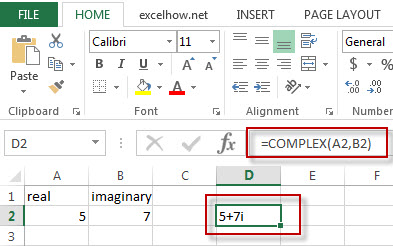# Excel Complex Function

This post will guide you how to use Excel COMPLEX function with syntax and examples in Microsoft excel.

### Description

The Excel COMPLEX function converts real and imaginary coefficients into a complex number.

The COMPLEX function is a build-in function in Microsoft Excel and it is categorized as an Engineering Function.

The COMPLEX function is available in Excel 2016, Excel 2013, Excel 2010, Excel 2007, Excel 2003, Excel XP, Excel 2000, Excel 2011 for Mac.

### Syntax

The syntax of the COMPLEX function is as below:

= COMPLEX (real_num,i_num,[suffix])

Where the COMPLEX function argument is:
Real_num -This is a required argument. The real coefficient of the complex number.
I_num -This is a required argument. The imaginary coefficient of the complex number.
Note:
If real_num value is not a numeric value, it will return a #VALUE! Error.
If i_num value is not a numeric value, it will return a #VALUE! Error.
If suffix is not either “i” nor “j”, it will return a #VALUE! Error.

### Example

The below examples will show you how to use Excel COMPLEX Function to convert coefficients into a complex number.

#1 =COMPLEX(A2,B2)